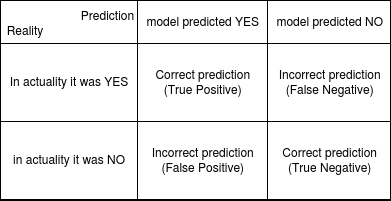# Understanding Precision and Recall

One of the ways to help our brain resolve confusing terminologies is by blogging about them. In the past, I have observed some promising results with this approach. This blog-post is intended to resolve my confusion between precision and recall.

Prof. Andrew Ng explains skewed classes with a great example -

In predicting a cancer diagnosis where 0.5% of the examples have cancer, we find our learning algorithm has a 1% error. However, if we were to simply classify every single example as DOES NOT HAVE CANCER, then our error would reduce to 0.5% even though we did not improve the algorithm.
The above example clearly describes the want of a better error metric. Ideally, we need a leap from (examples_correctly_classified)/(total_examples) to something more immune to skewed classes.

In enters the idea of positives and negatives. Concerning our classes, a positive is when our model tells YES to our example. Is this a cat? Yes! Is she eligible for a loan? Yes! Will it rain? Yes! In contrast, a negative is when our model tells NO to an example. Does he have cancer? No! Is this a Van Gogh? No! Does this name sound Indian? No!

After our model classifies examples as positives and negatives, we can neatly arrange all our model's predictions into 4 classes:Figure 1: False positives: Examples our model predicted as positive, it turned out to be False. False negatives: Examples our model predicted as negative, it turned out to be False.

Observing the above classes, we can draw a concrete conclusion for our cancer example. As we are predicting every example as a negative, we would perform horribly in model predicted NO → In actuality it was a YES condition (i.e. we have many false negatives).

With the above accuracy model, the classification game has shifted from increasing the number of correctly classified examples to reducing the number of false positives and false negatives. To make things more precise, we stress on two important questions -

• Precision - Out of all the examples model predicted as positive, how many were in fact positive?
• Recall - Out of all the examples which in reality were positive, how many were predicted as positive?
The above diagram can then be modified as follows: